## Abstract

In this paper, a simple microstrip patch antenna and a two-element E-plane coupled microstrip antenna array employing a defected ground structure are investigated. Without defected ground structure, the antenna has an impedance bandwidth of 675 MHz (6.78% at the center frequency of 9.955 GHz) and a gain of around 4.38 dB. The performance of the microstrip antenna in terms of impedance bandwidth, matching performance, gain and return loss can be improved by introducing a defect in the ground plane. As a result of which, bandwidth of 1.652 GHz (16.42% at the center frequency of 10.06 GHz) and a gain of 8.96 dB along with 5 different other operating bands are achieved. After integrating the microstrip antenna with the proposed defected ground structure, effective footprint of the antenna is reduced up to 66.95%. When integrated with proposed DGS array, the same antenna array structure shows miniaturization up to 78.97%. The proposed defected ground structure when compared to other techniques shows an exceptionally lower mutual coupling between two E-plane coupled microstrip antenna elements.

## Keywords

Microstrip patch antenna (MSPA) ; Defected ground structure (DGS) ; Electromagnetic bandgap (EBG) structure ; Surface waves ; Impedance bandwidth ; Side lobe level (SLL)

## 1. Introduction

The frequency response of various microwave components and circuits can be optimized by selecting the appropriated shape and size of the conductor lines. Since backside ground plane of these components, which is typically made up of metal sheet like copper, does not provide a degree of freedom in the design phase, defected ground structure (DGS) suggests an alternative solution to improve the characteristics of the microwave components that are based on microstrip lines and coplanar waveguides (CPW)  . Various slotted structures etched in the ground plane have been reported in   and  . Any modification or etching of the ground plane which alters its uniformity is generally called as “defect”  . It is positioned beneath a microstrip line or antenna and aligned for proper coupling to the microstrip line or antenna  . In DGS, the metallic ground plane is carefully etched for attaining the desired pass band, stop band and slow wave characteristics due to disturbance or perturbation of current distribution which in turn increases its effective capacitance and inductance   and  . This also influences the input impedance and current distribution of the antenna, thereby minimizing the size of the antenna with respect to the resonating frequency of the antenna  . This results in forbidden excitation and transmission of the EM waves through the dielectric layer  . Electromagnetic waves travelling in the DGS offer stop band and pass band characteristics over a range of frequencies, thereby leading to a slow wave structure  .

There are two main categories of DGS: one group may include a single defect or unit cell and the other may include a series of small defects in periodic or non-periodic manner  . DGS of various shapes along with their applications are discussed in  ,  ,  ,  ,  ,  ,   and  . This work presents a novel defected ground structure. The performance of microstrip antennas and their array can be enhanced by introducing the defects in the ground plane. So, one dimensional (1-D) EBG structure or DGS is designed and analyzed. First of all, its design principle is discussed and then its application in array design is considered. The performance of the proposed DGS is evaluated and compared to that of conventional microstrip antenna with an ordinary ground plane. The design procedure, measurement techniques, and analysis of bandgap for a simple square type DGS (both positive and negative) has already been published in  . In this paper, a compact MSPA having 5 operating bands is proposed.

This paper is organized as follows. Section II describes the conventional MSPA and its associated parameters. First of all, optimization is performed on the dimensions of conventional MSPA to obtain the desired resonating frequency, i.e. 10 GHz. Thereafter, dimensional analysis of proposed DGS unit cell structure is done in Section III. Section IV describes the procedure to obtain the proposed defected ground structure unit cell. Section V describes the parametric study of each DGS dimension unit and their impact on the antenna characteristics, which finally gives rise to optimized proposed DGS structure. Section VI includes the impact of different array configurations of proposed DGS structure on the antenna characteristics. In Section VII, comparison of proposed DGS is performed with other mutual coupling reduction techniques in perspective of microstrip antenna array designing.

## 2. Single patch antenna design

Rogers RT/duroid 5880 (εr  = 2.2, thickness, h = 1.6 mm, tan δ = 0.0009) is chosen as dielectric material. The dimensions of ground plane are 50 mm × 50 mm. The following formulas are used for calculating the dimensions of the microstrip patch. For an efficient radiator operating at frequency fr , placed on dielectric substrate of thickness h and permittivity Єr, the actual width is given by:

 $W={\frac {c}{2f_{r}{\sqrt {\frac {\left({\epsilon }_{r}+1\right)}{2}}}}}$
( 1)

Substituting velocity of light, c  = 3.00 × 108  m/s, εr  = 2.2 and fr  = 10 GHz, we get W  = 9.1224 mm. The effective dielectric constant (due to fringing effect) is:

 ${\epsilon }_{\mbox{eff}}={\frac {{\epsilon }_{r}+1}{2}}+$${\frac {{\epsilon }_{r}-1}{2}}{\left[1+12{\frac {h}{W}}\right]}^{-1/2}$
( 2)

Putting εr  = 2.2, W  = 9.122 mm and h  = 1.6 mm we get εeff=  3.18. Patch length extension at the two open ends due to fringing fields can be calculated as:

 $\Delta L=0.412h{\frac {\left({\epsilon }_{eff}+0.3\right)\left({\frac {W}{h}}+0.264\right)}{\left({\epsilon }_{eff}-0.258\right)\left({\frac {W}{h}}-0.8\right)}}$
( 3)

Replacing εeff , W, and h by their values, we get ΔL =  0.71537. Patch length can be calculated as:

 $L={\frac {c}{2f_{r}{\sqrt {{\epsilon }_{eff}}}}}-2\left(\Delta L\right)$
( 4)

Putting εeff  = 3.1824, we get L  = 6.9718 mm. The feed used is microstrip feed which is 21.51407 mm long and 2.325 mm wide. The final dimensions of the conventional microstrip antenna and feed are given in Table 1 . The impedance of the radiating patch can be approximately given as follows:

 $Z_{a}={\frac {90{\epsilon }_{r}{}^{2}}{{\epsilon }_{r}-1}}{\left({\frac {L}{W}}\right)}^{2}$
( 5)

Table 1. Design parameters of normal microstrip antenna after optimization.
Parameter Value
λo 30 mm
Frequency of operation, fo 10 GHz
Substrate Rogers RT/duroid 5880
Substrate thickness, h 1.6 mm
εr 2.2
W 9.122 mm
L 6.9718 mm
Wf 2.325 mm
Lf 21.514 mm

The impedance of the patch comes out to be 299.32Ω. Since we are using microstrip feed, so for better impedance matching between the radiating patch and feed, the length and width of the feed are taken to be 2.325 and 21.514 mm.

Fig. 1 shows an optimized conventional microstrip antenna above a non-defected ground plane which is designed to operate at around 10 GHz. Microstrip feed is used to excite the antenna. Since the return loss level and impedance bandwidth are affected with respect to the feeding location, the same is varied at a step of 0.25 mm starting from the extreme corner point moving towards the center of the microstrip patch or radiator. So, only that feeding point is selected where the values of return loss and impedance bandwidth is best. Here we are able to achieve the best results when the feed is used at extreme corner. Feed width (Wf ) is also considered for better impedance matching as it affects the impedance nature of the radiating structure. Table 2 shows the simulation results of conventional MSPA, showing very small value of %BW and gain.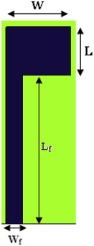Fig. 1. Conventional microstrip patch antenna.

Table 2. Simulation results for conventional MSPA.
S. No. Conventional MSPA Values S. No. Conventional MSPA Values
1. fr (GHz) 9.9550 5. BW 675 MHz
2. S11 (dB) −19.3287 6.  %BW 6.7875
3. VSWR 1.2422 7. FBR 156.945
4. Gain (dB) 4.3841

## 3. DGS unit cell configuration and integration with MSPA

Fig. 2 shows the proposed etched defected ground structure on the ground plane. Fig. 3 shows the alignment of proposed DGS with radiating patch. Dimensions of the proposed DGS unit cell are given in Table 3 . The proposed DGS structure consists of a metallic strip of 2 mm around the ground plane. It has two concentric square metallic patches in its center. The dimensions of inner square patch and outer square patch are s × s and of L1  × L1 respectively, where s = 10 mm and L1  = 20 mm as shown in Table 3 . Gap between both patches is 2 mm. It has a rectangular pattern having the thickness of 3 mm (= L1 –L2 ) on its both sides, each connected to the central outer square patch via two strips. The dimensions of these strips are dp  × dT . Designing of the DGS is done in such a way the more electric field gets trapped within the defect, thereby causing the capacitive effect, while the current flowing around the DGS gives rise to inductive effect, which in turn, results in resonant characteristics of a DGS. This causes a bandstop frequency response. Fig. 4 shows the return loss comparison of conventional MSPA (shown in Fig. 1 ) with that of MSPA with proposed DGS. It is evident from Fig. 4 that bandwidth has increased from 675 MHz to 1.6517 GHz and several other bands are also obtained. For conventional MSPA, only one band i.e. at 9.955 GHz (for S11 <-10 dB) was obtained, but after integrating with proposed DGS, frequency bands at 7.5976, 10.0601, 12.5976, 15.961 and 17.6276 GHz (for S11  <−10 dB) were also obtained. Hence, the MSPA with proposed DGS can be used for amateur radio, amateur satellite applications and other C and, Ku band applications.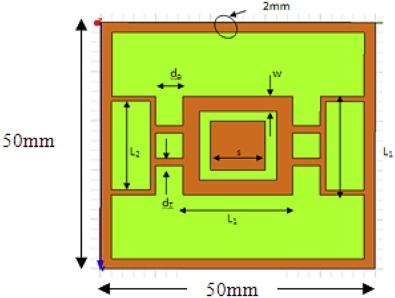Fig. 2. Schematic representation of one unit cell of the proposed DGS structure (50 mm × 50 mm).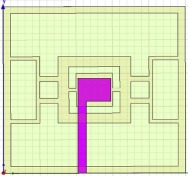Fig. 3. Simple microstrip antenna with proposed DGS structure.

Table 3. Dimensions of proposed unit cell DGS.
S. No. Parameter Value S. No. Parameter Value
1. L1 20 mm 5. S 10 mm
2. L2 18 mm 6. W 3 mm
3. dT 1.5 mm 7. Central patch dimensions (s × s) 10 mm × 10 mm
4. dp 5 mm 8. Corner strip 2 mm (shown in Fig. 2 )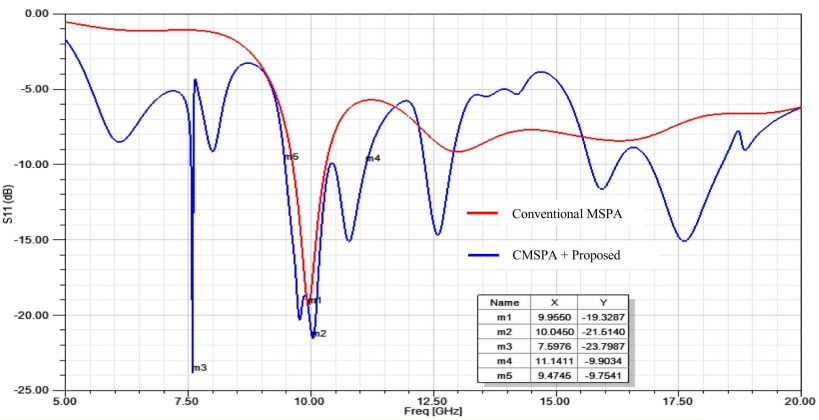Fig. 4. Schematic representation of one unit cell of the proposed DGS structure (50 mm × 50 mm).

Since we have obtained one lower resonating frequency (at 7.5976 GHz) as well, miniaturization up to 66.95% has been achieved. The gain at 10 GHz for both E and H-planes is plotted in Fig. 5a and b respectively. For simple MSPA, the gain of 4.3841 dB was observed, whereas after integrating with proposed DGS, gain becomes 8.96 dB. Here, a small rise in antenna efficiency from 1.0219 to 1.028 is also seen.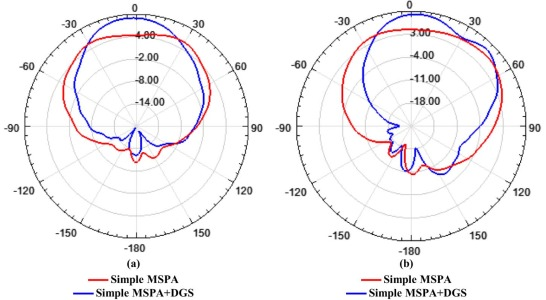Fig. 5. Variation of gain (in dB) of the simple MSPA and MSPA integrated with proposed DGS having 5 operating bands. (a) E-plane (b) H-plane at 10 GHz.

## 4. Effect of array of DGS and its array on antenna characteristics

In order to obtain better impedance matching, increasing the number of operating bands and bandwidth, same DGS pattern is scaled at different levels and etched in the ground plane. In Fig. 6 , a scaled version of the proposed DGS structure (shown in Fig. 2 ) is shown to obtain its 2 × 2 array. Since the thinnest line that can be etched with a precise lithographic technique is 0.1 mm (approx.), simulation up to 2 × 2 DGS array is done. In Fig. 7a and b , co-polarization and cross polarizations levels in the E and H planes of conventional MSPA and MSPA with proposed 2 × 2 DGS are compared. Simulation results of 2 × 2 array obtained from comparison between proposed DGS and conventional MSPA as shown in Fig. 7a in E-plane reveal that there is a decrement of 3 dB and 7.261 dB in co-polarization and cross-polarization levels, respectively. Also, the SLL (side lobe level) was 3 dB in conventional MSPA, but after integrating with DGS, it becomes 16.6372 dB. Similarly in H-plane shown in Fig. 7b , the co-polarization and cross-polarization levels decrease up to 2.63 dB and 3.5 dB, respectively, with SLL of 24.05 dB. There is a considerable decrease in the co-polarization and cross-polarization levels in E-plane as we made etched the ground plane in θ = 0° (or E-plane) direction and less changes are made in θ = 90° (or H-plane). Fig. 8 shows the return loss comparison results of 2 × 2 and 4 × 4 proposed DGS array with conventional MSPA.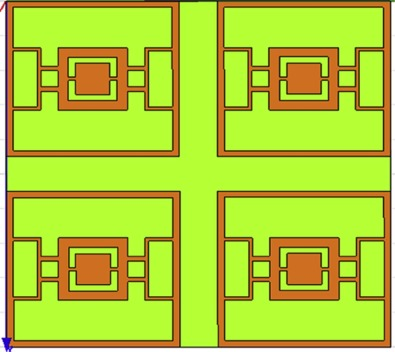Fig. 6. Array of proposed DGS integrated with MSPA for reducing mutual coupling level.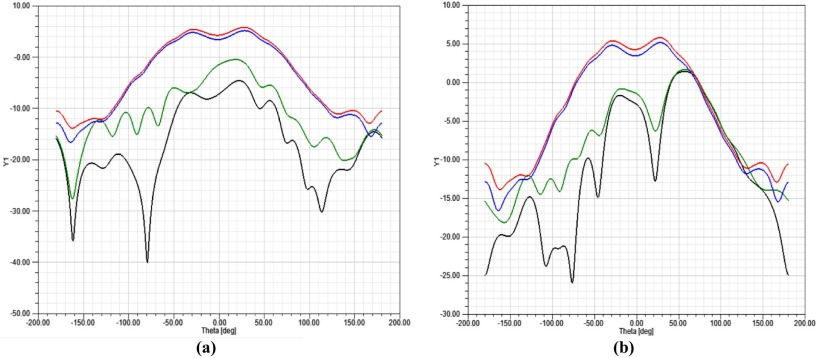Fig. 7. Radiation patterns of 2 × 2 DGS array at fr  = 7.6286 GHz. (A) E-plane pattern. (B) H-plane pattern. The radiation pattern shows that the MSPA is radiating efficiently and there is a decrement of 8 dB at θ = 0° in E-plane cross polarization levels.Conventional MSPA (co-pol)2 × 2 DGS (co-pol).Conventional MSPA (x-pol)2 × 2 DGS (x-pol).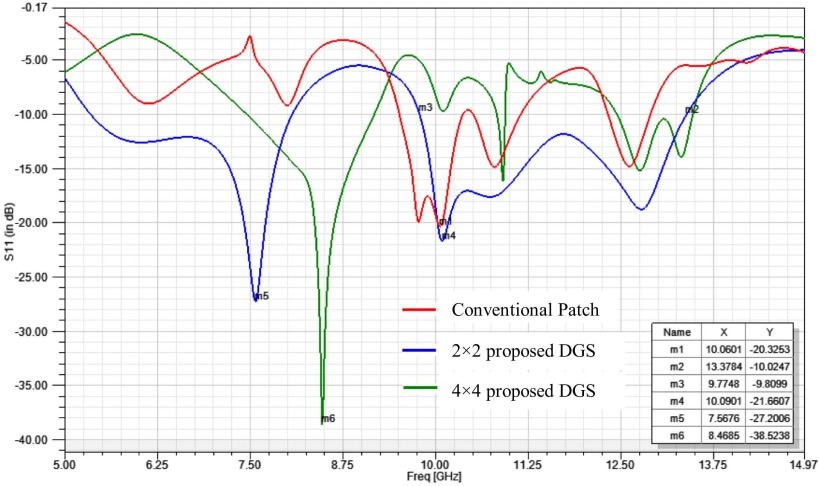Fig. 8. Comparison of 2 × 2 and 4 × 4 DGS array with conventional MSPA.

## 5. Comparison of proposed DGS with other approaches for mutual coupling reduction in microstrip array

The main drawback of E-plane coupled microstrip patch antenna arrays is that they possess the high excitation of surface waves. Because of this, strong mutual coupling exists among these patch antenna of the arrays. So it is instructive to investigate this effect in the conventional patch array, having the resonating frequency 10 GHz along with our proposed DGS and its array. Here, 4 mutual coupling reduction techniques, namely

• conventional MSPA,
• the substrate between the antenna is removed,
• cavity back MSPA, and
• MSPA with EBG structure in between. are simulated and compared. While comparing the above mentioned mutual coupling reduction techniques, substrate properties, antenna size and distance between them are kept constant.

Fig. 9 shows the MSPA array chosen to compare these techniques. Table 6 shows the effect of varying gap between the conventional patch antennas. While comparing these techniques, the distance between the two patches is kept 0.5λo . Fig. 10 shows the MSPA array in which substrate between the antennas is removed. Fig. 11 shows the cavity back mutual reduction technique. Fig. 12a and b shows the mushroom type EBG structure and its close view respectively. (Table 4 ).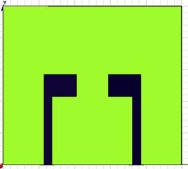Fig. 9. MSPA array chosen to compare the mutual coupling reduction techniques.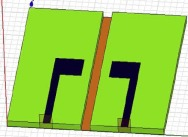Fig. 10. MSPA array having removed substrate in between them.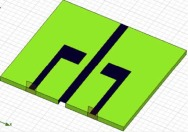Fig. 11. MSPA array showing cavity back mutual coupling reduction technique.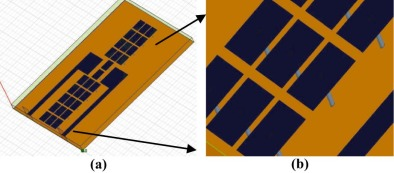Fig. 12. (a) MSPA array with mushroom type EBG structure in between them. (b) Close view of the mushroom-type EBG.

Table 4. Effect of distance between the patch antennas.
Normal MSPA array 0.35λo 0.4λo 0.5λo 0.6λo
fr (GHz) 9.7297 9.8949 10.045 9.939
S11 (dB) −15.209 −19.370 −18.296 −19.649
S21 (dB) −8.8796 −14.856 −25.154 −33.251
BW (GHz) 1.1862 0.9009 0.765 0.825

Fig. 13 shows return loss comparison of 3-lines mushroom-type EBG backed MSPA array with conventional MSPA array. It is evident from Table 7 that mutual reduction for 3-lines of mushroom-type EBG is about 9.39 dB, which is less when compared to proposed DGS and it 2 × 2 structure (shown in Table 8  and Table 9 ).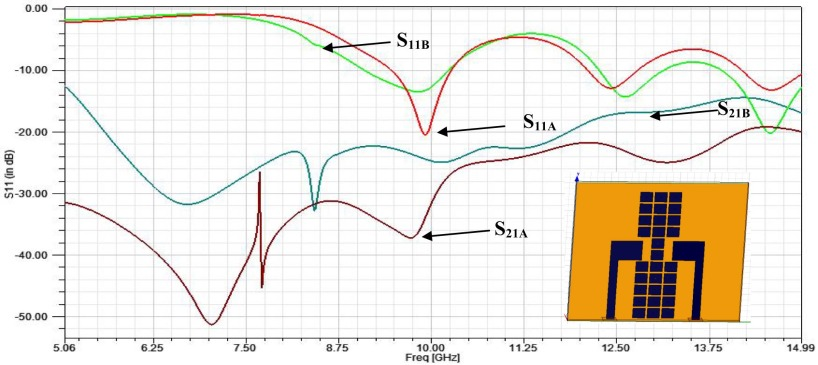Fig. 13. Comparison of S21 parameter of normal microstrip array with mushroom-type EBG (3 lines). Here ‘A’ is used for simple MSP array and ‘B’ for EBG.

Table 5  and Table 6 show the effect of cavity and substrate removal between the array elements. Table 7 shows the effect of mushroom-type EBG structure on the MSPA array. Table 8  and Table 9 show the effect of proposed DGS and its 2 × 2 array (as shown in Fig. 5 ) on the MSPA array. It is evident from Table 10 that proposed DGS antenna has highest mutual coupling reduction of about 23.71 dB, which is highest among all reduction techniques. Fig. 14 shows that miniaturization up to 78.973% can be achieved as we are getting one frequency band (6.111 GHz) below our resonant frequency band (i.e. 10 GHz). But, if we use the array of the same DGS, better results will be seen. Mutual coupling reduction of up to 36.11 dB can be achieved, which is again lowest among all approaches. For comparisons shown in Table 10 , the distance between the two antennas is taken as 0.5λo . In case of cavity backed and substrate removal, gap or cavity is taken to be 3 mm. As expected, in conventional antenna, the mutual coupling decreases as the separation between the antennas increases. But for the proposed DGS and its array, a contradictory phenomenon is observed as shown in Fig. 15 . (Table 5 , Table 6 , Table 7 , Table 8 , Table 9 , Table 10  and Table 11 ).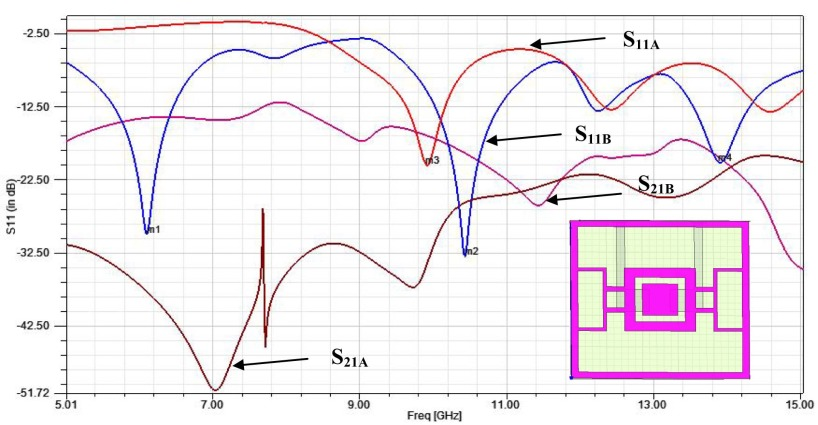Fig. 14. Comparison of S21 parameter of normal microstrip array with proposed DGS. Here ‘A’ is used for simple MSP array and ‘B’ for proposed DGS.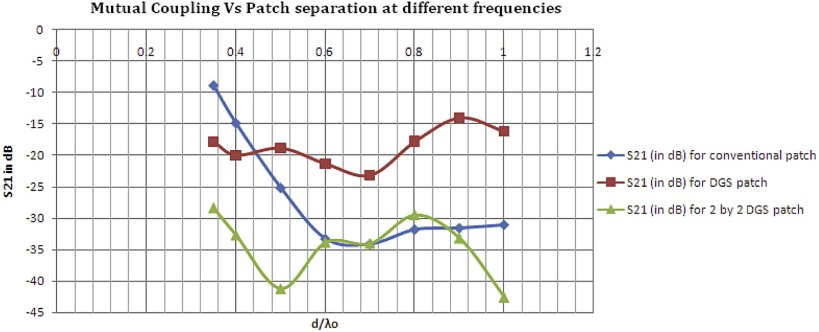Fig. 15. Mutual coupling vs. patch separation at the resonant frequency.

Table 5. Effect of cavity between the patch antennas.
Cavity Back d = 1 mm d = 2 mm d = 3 mm d = 4 mm
fr (GHz) 10.0601 10.00 9.9850 9.9700
S11 (dB) −17.9965 −18.7182 −19.00 −19.973
S21 (dB) −38.3343 −40.4254 −31.8196 −37.1151
BW (MHz) 765.8 825.8 810.8 855.9

Table 6. Effect of substrate removed between the patch antennas.
Substrate Removed d = 1 mm d = 2 mm d = 3 mm d = 4 mm
fr (GHz) 10.000 9.97 9.9099 10.015
S11 (dB) −19.0332 −19.2027 −20.4803 −19.9487
S21 (dB) −34.2871 −32.3325 −28.1368 −19.9487
Gain (dB) 5.4744 5.4722 5.2977 5.4409
BW (MHz) 795.8 810.8 810.8 780.8

Table 7. Effect of mushroom type EBG on MSPA array.
Substrate Removed 1 line 3 lines 5 lines
fr (GHz) 10.2252 9.8198 9.8949
S11 (dB) −15.2117 −13.4934 −23.508
S21 (dB) −14.8324 −23.9901 −34.5483
BW (GHz) 1.1261 1.1261 1.5816

Table 8. Effect of proposed defected ground structure for different distance between the antennas.
Substrate Removed 0.35λo 0.4λo 0.5λo 0.6λo
fr (GHz) 10.6607 10.6456 10.4354 10.3303
S11 (dB) −24.4717 −25.2230 −33.0166 −25.2955
S21 (dB) −17.8625 −19.9816 −48.8657 −21.3392
BW (GHz) 1.4264 1.3363 1.2162 1.2012

Table 9. Effect of 2 × 2 array of proposed defected ground structure for different distance between the antennas.
Substrate Removed 0.35λo 0.4λo 0.5λo 0.6λo
fr (GHz) 10.9910 11.2312 11.5315 11.9069
S11 (dB) −47.2128 −28.6775 −19.1814 −135638
S21 (dB) −28.3554 −32.7135 −61.2643 −33.8564
BW (GHz) 1.2162 1.2012 1.1562 1.0961

Table 10. Comparison of different mutual coupling reduction techniques.
Different Techniques Substrate removal Cavity backed EBG (5 layers) DGS DGS 2 × 2 array
Mutual coupling reduction (dB) 2.98 6.66 9.39 23.71 36.11

Table 11. Comparison with different other proposed defected ground structures.
Different Techniques Proposed Proposed DGS 2 × 2 array    
Mutual coupling reduction (dB) 23.71 36.11 18.28 2.528 17.52 20

## 6. Conclusion

In this work, a comprehensive investigation of defected ground structure, its various configurations and its impact on the MSPA characteristics is presented. We have seen that the conventional MSPA has very narrow bandwidth and it worked only in one band due to surface wave excitation and various other losses. So, it is integrated with the proposed defected ground structure to enhance its performance in terms of its widened impedance bandwidth, higher gain and return loss.

The conventional MSPA which resonates only at 9.9550 GHz, after integrating with DGS, starts resonating at 5 different frequencies, i.e. at 7.5976, 10.0601, 12.5976, 15.961 and 17.6276 GHz, keeping FBR nearly constant, which is shown in Fig. 11 . Peak gain of the antenna increases by 4.578 dB with the proposed DGS. It is also observed that the resonating frequency of the DGS antenna shifts downward when compared to conventional MSPA. This very small shift in resonating frequency is due to the slow wave effects of DGS. Due to this, miniaturization of about 66.95% can be achieved, which goes up to 78.973% after integrating MSPA array with 2 × 2 DGS array. Table 11 shows that the proposed DGS shows mutual coupling reduction of 23.71 dB which is 5.43 dB, 2.528 dB, 17.52, and 20 dB enhancement in comparison to the DGS presented in  ,  ,   and  respectively. Table 12 has accumulated all essential data for observing the effect of 2 × 2 and 4 × 4 DGS array on the MSPA closely. (Fig. 16 ).

Table 12. Simulation results for different 2 × 2 and 4 × 4 DGS array configurations.
DGS array fr (GHz) S11 (dB) FBR BW (GHz) BW (%) No. of bands E-field (dB) HPBW
2 × 2 7.6286 −28.774 195.03 2.6036 34.129 2 19.1001 52°
12.7167 −17.431 74.12 2.9439 23.15 18.1135 28°
4 × 4 7.6396 −15.758 70.278 0.3243 4.246 8 24.4145 33°
7.982 −15.903 49.444 0.1261 1.5798 16.8016 29°
8.7027 −42.811 59.715 0.8108 9.3074 14.6628 53°
9.9279 −17.103 104.06 0.1802 1.8150 9.2374 70°
10.7027 −17.848 51.279 0.1081 1.0100 12.5320 39°
12.8649 −13.657 88.66 0.5586 4.3420 9.4726 102°
13.5676 −11.522 1483.7 0.1982 1.4608 12.8615 29°
18.0901 −11.985 70.208 0.9730 5.3786 9.3290 21°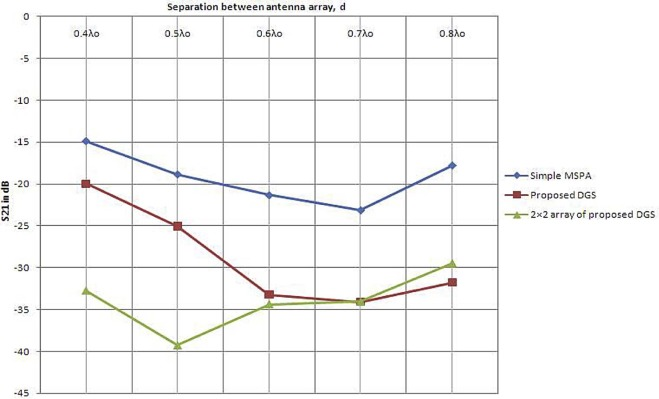Fig. 16. Comparison of S21 (in dB) of simple or conventional MSPA with and without proposed DGS and its array.

### Document informationPublished on 10/04/17

Licence: Other

### Document Score0

Views 21
Recommendations 0